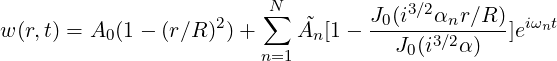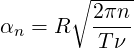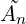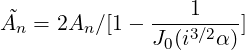### 11.3 Session file configuration

In the following the possible options are shown for the incompressible Navier-Stokes. The `Expansion` section for an incompressible flow simulation can be set as for other solvers regardless of the projection type. Here an example for a 3D simulation (for 2D simulations the specified fields would be just `u,v,p`).

1<EXPANSIONS>
2  <E COMPOSITE="C" NUMMODES="6" FIELDS="u,v,w,p" TYPE="MODIFIED" />
3</EXPANSIONS>

In case of a simulation using the Direct Solver we need to set `FIELDS=u,v` as the pressure expansion order will be automatically set to fulfil the inf-sup condition. Possible choices for the expansion `TYPE` are:

 Basis `TYPE` `Modal` `MODIFIED` `Nodal` `GLL_LAGRANGE` `Nodal SEM` `GLL_LAGRANGE_SEM`

#### 11.3.1 Solver Info

The following parameters can be specified in the `SOLVERINFO` section of the session file:

• `EqType`: sets the kind of equations we want to solve on the domain as:

Possible values are:

 Equations `EQTYPE` Dim. Projections Alg. Steady Stokes (SS) `SteadyStokes` All CG VCS Steady Oseen (SO) `SteadyOseen` All CG DS Unsteady Stokes (US) `UnsteadyStokes` All CG VCS Steady Linearised NS (SLNS) `SteadyLinearisedNS` All CG DS Unsteady Linearised NS (ULNS) `UnsteadyLinearisedNS` All CG VCS,DS Unsteady NS (UNS) `UnsteadyNavierStokes` All CG,CG-DG VCS
• `SolverType`: sets the scheme we want to use to solve the set of equations as
1<I PROPERTY="SolverType"  VALUE="VelocityCorrectionScheme"/>

Possible values are:

 Algorithm `SolverType` Dimensions Projections Velocity Correction Scheme (VCS) `VelocityCorrectionScheme` 2D, Quasi-3D, 3D CG, CG-DG VCS with weak pressure `VCSWeakPressure` 2D, Quasi-3D, 3D CG, CG-DG Direct solver `CoupledLinearisedNS` 2D, Quasi-3D CG
• `Driver`: this specifies the type of problem to be solved:

 Driver Description Dimensions Projections `Standard` Time integration of the equations All CG, DG `SteadyState` Steady-state solver (see Sec. 11.1.4) All CG, DG
• `Projection`: sets the Galerkin projection type as
1<I PROPERTY="Projection" VALUE="Continuous"/>

Possible values are:

 Galerkin Projection `Projection` Dimensions Equations Algorithms Continuous (CG) `Continuous` All All All Discontinuous (DG) `DisContinuous` All ... ... Mixed CG and DG (CG-DG) `Mixed_CG_Discontinuous` 2D,3D just UNS VCS-substepping
• `TimeIntegrationMethod`: sets the time integration method as
1<I PROPERTY="TimeIntegrationMethod" VALUE="IMEXOrder2"/>

Possible values are

 Time-Integration Method `TimeIntegrationMethod` Dimensions Equations Projections IMEX Order 1 `IMEXOrder1` all US, UNS CG IMEX Order 2 `IMEXOrder2` all US, UNS CG IMEX Order 3 `IMEXOrder3` all US, UNS CG Backward Euler `BackwardEuler` all US, UNS CG-DG BDF Order 1 `BDFImplicitOrder1` all US, UNS CG-DG BDF Order 2 `BDFImplicitOrder2` all US, UNS CG-DG
• `Extrapolation`: Specify the extrapolation method (standard or substepping) to be used in velocity correction scheme. Essentially this activates the sub-stepping routine which requires the mixed CG-DG projection
1<I PROPERTY="Extrapolation" VALUE="SubStepping"/>

Possible values are `SubStepping` or `Standard` with “Standard” being the default value if nothing is specifiied.

• `SubStepIntScheme`: choose the substep DG time integration scheme so that a different order schems can be used as compared to the overal time integraiton scheme.
1<I PROPERTY="SubStepIntScheme" VALUE="RungeKutta2\_ImprovedEuler"/>

Possible values are

 Time-Integration Method `SubStepIntScheme` Forward Euler `ForwardEuler` RK 2 `RungeKutta2_ImprovedEuler`

This option is useful if you wish to use an overall scheme that is first order accurate for example with TimeIntegrationMethod as BDFImplicitOrder1 but using a second order RungeKutta2_ImprovedEuler for greater stability in the substep.

• `GlobalSysSoln`: sets the approach we use to solve the the linear systems of the type Ax = b appearing in the solution steps, such as the Poisson equation for the pressure in the splitting-scheme. It can be set as
1<I PROPERTY="GlobalSysSoln" VALUE="IterativeStaticCond"/>

Possible values are

 System solution `GlobalSysSoln` Parallel Direct Solver (DS) `DirectFull` just quasi-3D DS with Static Condensation `DirectStaticCond` just Quasi-3D DS with Multilevel Static Condensation `DirectMultiLevelStaticCond` just Quasi-3D Iterative Solver (IS) `IterativeFull` just Quasi-3D IS with Static Condensation `IterativeStaticCond` quasi-3D IS with Multilevel Static Condensation `IterativeMultiLevelStaticCond` quasi-3D

Default values are `DirectMultiLevelStaticCond` in serial and `IterativeStaticCond` in parallel.

• `SmoothAdvection`: activates a stabilization technique which smooths the advection term using the pressure inverse mass matrix. It can be used just in combination with nodal expansion basis for efficiency reasons.
• `SpectralVanishingViscosity`: activates a stabilization technique which increases the viscosity on the modes with the highest frequencies.
1<I PROPERTY="SpectralVanishingViscosity" VALUE="True"/>

In a Quasi-3D simulation, this will affect both the Fourier and the spectral/hp expansions. To activate them independently, use `SpectralVanishingViscositySpectralHP` and `SpectralVanishingViscosityHomo1D`.

There are three spectral vanishing viscosity kernels available:

 SVV Kernel `SpectralVanishingViscosity` Exponential Kernel `True` Power Kernel `PowerKernel` DG Kernel `DGKernel`

The Exponential kernel is based on the work of Maday et al. , its extension to 2D can be found in . A diffusion coefficient can be specified which defines the base magnitude of the viscosity; this parameter is scaled by h∕p. SVV viscosity is activated for expansion modes greater than the product of the cut-off ratio and the expansion order. The Power kernel is a smooth function with no cut-off frequency; it focusses on a narrower band of higher expansion modes as the polynomial order increases. The cut-off ratio parameter for the Power kernel corresponds to the power ratio, see Moura et al. . The DG-Kernel is an attempt to match the dissipation of CG-SVV to DG schemes of lower expansion orders. This kernel does not require any parameters although the diffusion coefficient can still be modified.

• `DEALIASING`: activates the 3/2 padding rule on the advection term of a Quasi-3D simulation.
1<I PROPERTY="DEALIASING" VALUE="True"/>
• `SPECTRALHPDEALIASING`: activates the spectral/hp dealiasing to stabilize the simulation. This method is based on the work of Kirby and Sherwin .
1<I PROPERTY="SPECTRALHPDEALIASING" VALUE="True" />

#### 11.3.2 Parameters

The following parameters can be specified in the `PARAMETERS` section of the session file:

• `TimeStep`: sets the timestep for the integration in time formula.
• `NumSteps`: sets the number of time-steps.
• `IO_CheckSteps`: sets the number of steps between successive checkpoint files.
• `IO_InfoSteps`: sets the number of steps between successive info stats are printed to screen.
• `Kinvis`: sets the cinematic viscosity coefficient formula.
• `SubStepCFL`: sets the CFL safety limit for the sub-stepping algorithm (default value = 0.5).
• `MinSubSteps`: perform a minimum number of substeps in sub-stepping algorithm (default is 1).
• `MaxSubSteps`: perform a maxmimum number of substeps in sub-stepping algorithm otherwise exit (default is 100).
• `SVVCutoffRatio`: sets the ratio of Fourier frequency not affected by the SVV technique (default value = 0.75, i.e. the first 75% of frequency are not damped).
• `SVVDiffCoeff`: sets the SVV diffusion coefficient (default value = 0.1 (Exponential and Power kernel), 1 (DG-Kernel)).
• `IO_CFLWriteFld`: sets a treshold value for the CFL number. If CFL exceeds this value, the flow field is written to file (only once). This is useful for debugging purposes, allowing to visually inspect a flow field that is becoming unstable.
• `IO_CFLWriteFldNumSteps`: sets the number of timesteps after which `IO_CFLWriteFld` becomes operational. This avoids writing the flow field at the beginning of a simulation when initialising a new geometry.

#### 11.3.3 Womersley Boundary Condition

It is possible to define the time-dependent Womersley velocity profile for pulsatile flow in a pipe. The modulation of the velocity profile is based on the desired peak or centerline velocity which can be represented by a Fourier expansion Umax = A(ωn)ent where A are the Fourier modes and ω the frequency. The womersely solution is then defined as:where the womersley number α is defined:and(n = 1 : N)are the Fourier coefficients scaled in the following way:The Womersley velocity profile is implemented in the following way:

1<REGION REF="0">
2    <D VAR="u" USERDEFINEDTYPE="Womersley:WomParams.xml" VALUE="0" />
3    <D VAR="v" USERDEFINEDTYPE="Womersley:WomParams.xml" VALUE="0" />
4    <D VAR="w" USERDEFINEDTYPE="Womersley:WomParams.xml" VALUE="0" />
5    <N VAR="p" USERDEFINEDTYPE="H" VALUE="0" />
6</REGION>

A file containing the Fourier coefficients, Ã, must be in the directory where the solver is called from. The name of the file is defined by the string given in the attribute `USERDEFINEDTYPE` after the “:” and contains the real and imaginary coefficients. This file has the format

1<NEKTAR>
2  <WOMERSLEYBC>
3    <WOMPARAMS>
5      <W PROPERTY="Period" VALUE="1.0" />
6      <W PROPERTY="axisnormal" VALUE="0.0,0.0,1.0" />
7      <W PROPERTY="axispoint" VALUE="0.0,0.0,0.0" />
8    </WOMPARAMS>
9
10    <FOURIERCOEFFS>
11      <F ID="0"> 0.600393641193,    0.0               </F>
12      <F ID="1"> -0.277707172935,   0.0767582715413   </F>
13      <F ID="2"> -0.0229953131146,  0.0760936232478   </F>
14      <F ID="3"> 0.00858135174058,  0.017089888642    </F>
15      <F ID="4"> 0.0140332527651,   0.0171575122496   </F>
16      <F ID="5"> 0.0156970122129,   -0.00547357750345 </F>
17      <F ID="6"> 0.00473626554238,  -0.00498786519876 </F>
18      <F ID="7"> 0.00204434981523,  -0.00614566561937 </F>
19      <F ID="8"> -0.000274697215201, 0.000153571881197 </F>
20      <F ID="9"> -0.000148037910774, 2.68919619581e-05 </F>
21    </FOURIERCOEFFS>
22  </WOMERSLEYBC>
23</NEKTAR>

Each value of Ã is provided in the `FOURIERCOEFFS` section and provided as separate entries containing the real and imaginary components, i.e. the mean component provided above is 0.600393641193,0.0.

Similarly in the `WOMPARAMS` section the key parameters of the boundary condition are also provided as:

• `RADIUS` is the radius of the boundary.
• `PERIOD` is the cycle time period,
• `AXISNORMAL` defines the normal direction to the boundary,
• `AXISPOINT` defines a coordinate in the boundary centre,

#### 11.3.4 Forcing

##### 11.3.4.1 MovingBody

Note: This force type is only supported for the Quasi-3D incompressible Navier-Stokes solver.

This force type allows the user to solve the interaction system of an incompressible fluid flowing past a flexible moving bodies  . By this forcing function, one can eliminate the difficulty of moving mesh by using body-fitted coordinates, so that an additional acceleration term(i.e., forcing term) is introduced to the momentum equations by the non-inertial transform from the deformed and moving coordinate system to non-deformed and stationary one.

1<FORCE TYPE="MovingBody">
2</FORCE>

Available options of the motion type for the moving body include free, constrained and forced vibrations, which can be specified in the `SOLVERINFO` section. The free type of motion allows the body to move in both streamwise and crossflow directions, while the constrained type limits the motion only in the crossflow direction. For the forced type, the vibration profiles of the body should be specified as a given function or read from input file in `MovingBody` section. For example:

1<SOLVERINFO>
3    <I PROPERTY="SolverType"            VALUE="VelocityCorrectionScheme" />
4    <I PROPERTY="EvolutionOperator"     VALUE="SkewSymmetric"            />
5    <I PROPERTY="Projection"            VALUE="Galerkin"                 />
6    <I PROPERTY="GlobalSysSoln"         VALUE="DirectStaticCond"         />
7    <I PROPERTY="TimeIntegrationMethod" VALUE="IMEXOrder2"               />
8    <I PROPERTY="HOMOGENEOUS"           VALUE="1D"                       />
9    <I PROPERTY="USEFFT"                VALUE="FFTW"                     />
10    <I PROPERTY="VibrationType"         VALUE="FREE"                     />
11</SOLVERINFO>

A moving body type boundary condition should be specified in `BOUNDARYCONDITIONS` for the velocities on the moving body,

1<BOUNDARYCONDITIONS>
2    <REGION REF="0">
3        <D VAR="u" USERDEFINEDTYPE="MovingBody" VALUE="0" />
4        <D VAR="v" USERDEFINEDTYPE="MovingBody" VALUE="0" />
5        <D VAR="w" VALUE="0" />
6        <N VAR="p" USERDEFINEDTYPE="H" VALUE="0" />
7     </REGION>
8</BOUNDARYCONDITIONS>

For the simulation of low mass ratio, there is an option to activate fictitious mass method for stabilizing explicit coupling between the fluid solver and structural dynamic solver. Here we need to specify the values of fictitious mass and damping in `PARAMETERS`, for example,

1<SOLVERINFO>
2    <I PROPERTY="FictitiousMassMethod"    VALUE="True" />
3</SOLVERINFO>
4<PARAMETERS>
5    <P> FictDamp      = 1000    </P>
6    <P> FictMass       = 1.5    </P>
7</PARAMETERS>

A filter called `MovingBody` is encapsulated in this module to evaluate the aerodynamic forces along the moving body surface. The forces for each computational plane are projected along the Cartesian axes and the pressure and viscous contributions are computed in each direction.

The following parameters are supported:

 Option name Required Default Description `OutputFile` ✗ `session` Prefix of the output filename to which the forces are written. `Frequency` ✗ 1 Number of timesteps after which output is written. `Boundary` ✓ - Boundary surfaces on which the forces are to be evaluated.

To enable the filter, add the following to the `FORCE` tag::

1  <FORCE TYPE="MovingBody">
2      <PARAM NAME="OutputFile">DragLift</PARAM>
3      <PARAM NAME="OutputFrequency">10</PARAM>
4      <PARAM NAME="Boundary"> B </PARAM>
5  </FORCE>

During the execution a file named `DragLift.fce` will be created and the value of the aerodynamic forces on boundary 0, defined in the `GEOMETRY` section, will be output every 10 time steps.evaluates the aerodynamic forces along the moving body surface. The forces for each computational plane are projected along the Cartesian axes and the pressure and viscous contributions are computed in each direction.

Also, to use this module a `MAPPING` needs to be specified, as described in section 11.6. In the case of free and constrained motion presented here, the functions defined by the mapping act as initial conditions. Also, when using the MovingBody forcing, it is not necessary to set the `TIMEDEPENDENT` property of the mapping.

#### 11.3.5 Filters

The following filters are supported exclusively for the incompressible Navier-Stokes solver. Further filters from section 3.4 are also available for this solver.

• `Aerodynamic forces` (section 3.4.2)
• `Kinetic energy and enstrophy` (section 3.4.10)
• `Modal energy` (section 3.4.11)
• `Moving body` (section 3.4.12)
• `Reynolds stresses` (section 3.4.15)